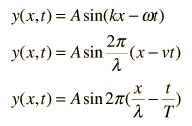# How can I plot time-dependent wave-functions?

I am trying to plot the wave-function of free particles as a function of x and time. Can someone explain or reference a site which explains how.

--Thank you

RaulTheUCSCSlug
Gold Member
So for this, it will be helpful if you understand a bit of multivariable calculus. Since the plot will not only be a function of time, but also a function of space (x,t) so your function now has two variables. We know this for a fact, because we encounter it in our daily lives. (at least we perceive it to be such a fact) Now looking at standard equation of a wave :We see how a function might change as x, and t changes. But much of the time, one parameter may seem rather arbitrary, and that parameter may be time.

I know that I am getting a bit off topic, but use the standard equations of a wave to guide you on what you want to plot. I believe Wolfram alpha has the ability to plot functions of several parameters if I am not mistaken. Experiment, and have a little fun with it. :)

So for this, it will be helpful if you understand a bit of multivariable calculus. Since the plot will not only be a function of time, but also a function of space (x,t) so your function now has two variables. We know this for a fact, because we encounter it in our daily lives. (at least we perceive it to be such a fact) Now looking at standard equation of a wave :We see how a function might change as x, and t changes. But much of the time, one parameter may seem rather arbitrary, and that parameter may be time.

I know that I am getting a bit off topic, but use the standard equations of a wave to guide you on what you want to plot. I believe Wolfram alpha has the ability to plot functions of several parameters if I am not mistaken. Experiment, and have a little fun with it. :)

Raul,

Thanks, and yeah I have been trying to plot the Solution to the Schrödinger equation for a free particle using Wolfram they have an interactive option where you can play around with the wave. However when plotting
$$\Psi(x,t)=e^{ikx-wt}$$
and I have x fixed, the real and imaginary components do not oscillate vertically with time. Maybe Python will do the trick?

--Freddy Cisneros

RaulTheUCSCSlug
Gold Member
Raul,

Thanks, and yeah I have been trying to plot the Solution to the Schrödinger equation for a free particle using Wolfram they have an interactive option where you can play around with the wave. However when plotting
$$\Psi(x,t)=e^{ikx-wt}$$
and I have x fixed, the real and imaginary components do not oscillate vertically with time. Maybe Python will do the trick?

--Freddy Cisneros

Damn, yeah maybe python can do the trick, or C. But that goes beyond the realm of my knowledge.

Damn, yeah maybe python can do the trick, or C. But that goes beyond the realm of my knowledge.

Thanks Raul, I'll look into it a little deeper.

--Freddy Cisneros

•RaulTheUCSCSlug